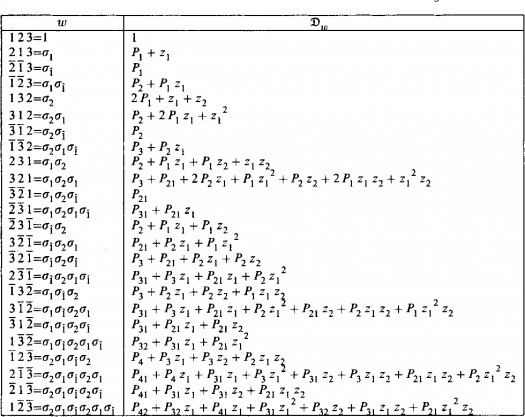# What Do You Know About Schubert Polynomials?

Approved & Edited by ProProfs Editorial Team
The editorial team at ProProfs Quizzes consists of a select group of subject experts, trivia writers, and quiz masters who have authored over 10,000 quizzes taken by more than 100 million users. This team includes our in-house seasoned quiz moderators and subject matter experts. Our editorial experts, spread across the world, are rigorously trained using our comprehensive guidelines to ensure that you receive the highest quality quizzes.
A
Community Contributor
Quizzes Created: 145 | Total Attempts: 38,511
Questions: 10 | Attempts: 129SettingsSchubert polynomials are speculations of Schur polynomials that speak to cohomology classes of Schubert cycles in flag or hail assortments.
In mathematics, there are many variations, which include double Schubert polynomials, quantum Schubert polynomials, and universal Schubert polynomials.
If you'd like to know more about Schubert polynomials, take the short quiz below to assess your knowledge.

• 1.

### Schubert polynomials are generalizations of Schur polynomials that represent which of these?

• A.

Trihomology classes

• B.

Dihomology classes

• C.

Homology classes

• D.

Teramology classes

C. Homology classes
Explanation
Schubert polynomials are generalizations of Schur polynomials, which are polynomials that represent homology classes. Homology classes are fundamental objects in algebraic topology that capture the properties and structure of spaces. Schubert polynomials provide a way to study and compute these homology classes in a more general setting. Therefore, the correct answer is homology classes.

Rate this question:

• 2.

### Who introduced Schubert polynomials?

• A.

Richard and Stanley

• B.

Lascoux and Schutzenberger

• C.

Lascoux and Richard

• D.

Stanley and Hermaine

B. Lascoux and Schutzenberger
Explanation
Lascoux and Schutzenberger introduced Schubert polynomials.

Rate this question:

• 3.

### After who are Schubert polynomials named?

• A.

Hermaine Schubert

• B.

Hermanne Schubert

• C.

Herman Schubert

• D.

Henry Schubert

B. Hermanne Schubert
Explanation
Schubert polynomials are named after Hermanne Schubert. The spelling of her name is "Hermanne" and not "Hermaine" or "Herman" or "Henry". Therefore, the correct answer is Hermanne Schubert.

Rate this question:

• 4.

### Which of the following does Schubert polynomials have?

• A.

Negative coefficient

• B.

Positive coefficient

• C.

Neutral coefficient

• D.

The coefficient depends on the value

B. Positive coefficient
Explanation
Schubert polynomials have positive coefficients. This means that each term in the polynomial has a positive coefficient, indicating that the polynomial is always positive or non-negative.

Rate this question:

• 5.

### Double Schubert polynomials are polynomials in what?

• A.

1 infinite set of variables

• B.

2 infinite sets of variables

• C.

3 infinite sets of variables

• D.

4 infinite set of variables

• E.

No infinite set of variables

B. 2 infinite sets of variables
Explanation
Double Schubert polynomials are polynomials in two infinite sets of variables. These polynomials are used in algebraic geometry and combinatorics to study the intersection theory on Grassmannians and flag varieties. The two infinite sets of variables correspond to the Schubert cells and the opposite Schubert cells, which are important objects in the study of flag varieties. The double Schubert polynomials encode information about the intersection numbers of these cells and have applications in representation theory and algebraic geometry.

Rate this question:

• 6.

### Which of these individuals put forth a conjectural rule for their coefficients?

• A.

Sara Billey

• B.

Richard P. Stanley

• C.

Sergey Formin

• D.

Lasoux

B. Richard P. Stanley
Explanation
Richard P. Stanley is the correct answer because he is known for his work in algebraic combinatorics and has made significant contributions to the field. In particular, he has put forth a conjectural rule for the coefficients of certain combinatorial polynomials, which suggests a relationship between various combinatorial objects. This conjecture has been influential in the study of combinatorial structures and has led to further research and developments in the field.

Rate this question:

• 7.

### Who described the history of Schubert polynomials?

• A.

Lascoux

• B.

Jockush

• C.

Sergey

• D.

Hermaine

A. Lascoux
Explanation
Lascoux is the correct answer because they are the one who described the history of Schubert polynomials.

Rate this question:

• 8.

### In which year was the history of Schubert polynomials described?

• A.

1995

• B.

1996

• C.

1997

• D.

1998

A. 1995
Explanation
The history of Schubert polynomials was described in the year 1995.

Rate this question:

• 9.

### What do we call the Schubert polynomials that can be seen as a generating function over certain combinatorial objects?

• A.

Pipe dreams or rc-graphs

• B.

Ripe dreams or rd-graphs

• C.

Side dreams or 3d-graphs

• D.

Side dreams or 4s-graphs

A. Pipe dreams or rc-graphs
Explanation
Pipe dreams or rc-graphs are called the Schubert polynomials that can be seen as a generating function over certain combinatorial objects.

Rate this question:

• 10.

### Fomin, Gelfand, and Postnikov introduced quantum Schubert polynomials in which year?

• A.

1997

• B.

1996

• C.

1995

• D.

1994

A. 1997
Explanation
Fomin, Gelfand, and Postnikov introduced quantum Schubert polynomials in 1997.

Rate this question:

Related TopicsBack to top